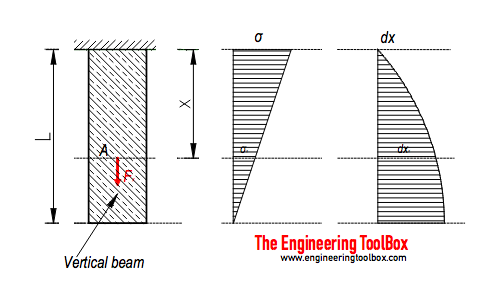Engineering ToolBox - Resources, Tools and Basic Information for Engineering and Design of Technical Applications!

# Weight of Beams - Stress and Strain

## Stress and deformation of vertical beams due to own weight.### Axial Force

The axial force acting in a cross sectional area in distance x in a vertical beam due to it's own weight - can be calculated as

Fx = γ  A (L - x)                                    (1)

where

Fx = axial force in distance x (N)

y = specific weight - unit volume weight (N/m3)

A = cross-sectional area (m2)

L = length of beam (m)

x = distance (m)

The specific weight can be expressed as

y = ρ g                                          (2)

where

ρ = density of beam (kg/m3)

g = acceleration of gravity (9.81 m/s2)

(1) can with (2) be modified to

Fxρ g  A (L - x)                            (2b)

### Axial Stress

The axial stress at a distance x can be calculated as

σx = Fx / A

= γ (L - x)

=  ρ g (L - x)                              (3)

where

σx = axial stress (Pa, N/m2)

Note! - the cross-sectional area is irrelevant.

The axial stress at distance x = L

σx=L = γ (L - L)

= ρ g (L - L)

= 0                                    (3a)

The axial stress at distance x = 0

σx=0 = γ (L - 0)

= ρ g (L - 0)

= γ L

= ρ g L                               (3b)

### Axial Deformation

The axial deformation at distance x can be calculated as

dxx = y x (2 L - x) / (2 E)

=   ρ g x (2 L - x) / (2 E)                           (4)

where

dx = deformation (m)

E = modulus of elasticity (N/m2)

The axial deformation at distance x = L

dxx=L = y L2 / (2 E)

= ρ g L2 / (2 E)                                (4a)

The axial deformation at distance x = 0

dxx=0 = 0                                    (4b)

### Example - Stress and Axial Deformation of a Vertical Steel Rod

A 45 m long steel rod with density 7280 kg/m3 and cross-sectional area 0.1 m2 is mounted as indicated in the figure above.

The maximum force acting in the rod at distance x = 0 m can be calculated with (1b)

Fx=0 (7280 kg/m3) (9.81 m/s2) (0.1 m2) ((45 m) - (0 m))

= 321376 N

= 321 kN

The maximum axial stress in the rod at distance x = 0 m can be calculated (3b)

σx=0 = (7280 kg/m3) (9.81 m/s2) (45 m)

= 3213756 N/m2 (Pa)

= 3.2 MPa

The modulus of elasticity for the steel rod is 200 GPa (200 109 N/m2).  The axial deformation at distance x = 45 m can be calculated with (4a)

dxx=45 = (7280 kg/m3) (9.81 m/s2) (45 m)2 / (2 (200 109 N/m2))

= 0.00036 m

= 0.4 mm

## Related Topics

• ### Beams and Columns

Deflection and stress, moment of inertia, section modulus and technical information of beams and columns.
• ### Mechanics

Forces, acceleration, displacement, vectors, motion, momentum, energy of objects and more.

## Related Documents

• ### American Standard Beams - S Beam

American Standard Beams ASTM A6 - Imperial units.
• ### American Wide Flange Beams

American Wide Flange Beams ASTM A6 in metric units.
• ### Area Moment of Inertia - Typical Cross Sections I

Typical cross sections and their Area Moment of Inertia.
• ### Beam Loads - Support Force Calculator

Calculate beam load and supporting forces.

• ### British Universal Columns and Beams

Properties of British Universal Steel Columns and Beams.
• ### Cantilever Beams - Moments and Deflections

Maximum reaction forces, deflections and moments - single and uniform loads.
• ### Euler's Column Formula

Calculate buckling of columns.
• ### HE-A Steel Beams

Properties of HE-A profiled steel beams.
• ### Normal Flange I-Beams

Properties of normal flange I profile steel beams.

Weight rating of threaded hanger rods.

• ### W-Beams - American Wide Flange Beams

Dimensions of American Wide Flange Beams ASTM A6 (or W-Beams) - Imperial units.
• ### Wood Beams - Strength of Material

Bending and compression strength of wood species used in beams.
• ### Young's Modulus, Tensile Strength and Yield Strength Values for some Materials

Young's Modulus (or Tensile Modulus alt. Modulus of Elasticity) and Ultimate Tensile Strength and Yield Strength for materials like steel, glass, wood and many more.

## Engineering ToolBox - SketchUp Extension - Online 3D modeling!

Add standard and customized parametric components - like flange beams, lumbers, piping, stairs and more - to your Sketchup model with the Engineering ToolBox - SketchUp Extension - enabled for use with older versions of the amazing SketchUp Make and the newer "up to date" SketchUp Pro . Add the Engineering ToolBox extension to your SketchUp Make/Pro from the Extension Warehouse !

We don't collect information from our users. More about

## Citation

• The Engineering ToolBox (2016). Weight of Beams - Stress and Strain. [online] Available at: https://www.engineeringtoolbox.com/weight-beam-stress-strain-d_1937.html [Accessed Day Month Year].

Modify the access date according your visit.

9.27.11